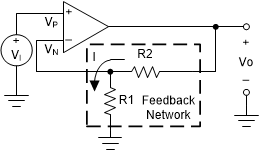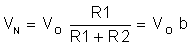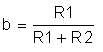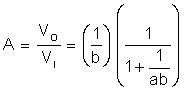SLOA011B January   2018  – July 2021

1. 1Introduction
2. 2Non-Inverting Amplifier
3. 3Inverting Amplifier
4. 4Simplified Op Amp Circuit Diagram
5. 5Op Amp Specifications
6. 6References
7. 7Glossary
8. 8Revision History

# 2 Non-Inverting Amplifier

An ideal op amp by itself is not a very useful device since any finite input signal would result in infinite output. By connecting external components around the ideal op amp, we can construct useful amplifier circuits. Figure 2-1 shows a basic op amp circuit, the non-inverting amplifier. The triangular gain block symbol is used to represent an ideal op amp. The input terminal marked with a + (Vp) is called the non-inverting input; – (Vn) marks the inverting input.Figure 2-1 Non-Inverting Amplifier

To understand this circuit we must derive a relationship between the input voltage, Vi, and the output voltage, Vo.

Remembering that there is no loading at the input,

Equation 11. Vp = VI

The voltage at Vn is derived from Vo via the resistor network, R1 and R2, so that,

Equation 12.where,

Equation 13.The parameter b is called the feedback factor because it represents the portion of the output that is fed back to the input.

Recalling the ideal model,

Equation 14. Vo = aVσ = a(Vp - Vn)

Substituting,

Equation 15. Vo = a(Vi - bVo)

and collecting terms yield,

Equation 16.This result shows that the op amp circuit of Figure 2-1 is itself an amplifier with gain A. Since the polarity of Vi and VO are the same, it is referred to as a non-inverting amplifier.

A is called the close loop gain of the op amp circuit, whereas a is the open loop gain. The product ab is called the loop gain. This is the gain a signal would see starting at the inverting input and traveling in a clockwise loop through the op amp and the feedback network.